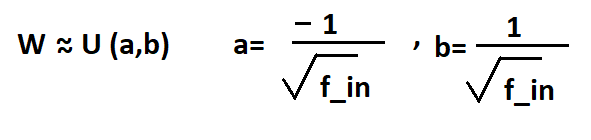top of pageSearch

# Weight Initialization Techniques-What best works for you

Weights are responsible for connection between the units, in neural networks, these can be initialized randomly and then get updated in back propagation in order to reduce the loss.

Few important things to keep in mind before initializing weights:

1) Weights should be small but not too small as it gives problems like vanishing gradient problem( vanish to 0). That is it will take forever to converge to global minima.

Weights can’t be too high as gives problems like exploding Gradient problem(weights of the model explode to infinity), which means that a large space is made available to search for global minima hence convergence becomes slow.

To prevent the gradients of the network’s activations from vanishing or exploding, we need to have following rules:

1. The mean of the activations should be zero.

2. The variance of the activations should stay the same across every layer.

2) Weights should be different, these can’t be same as it gives problems like all neurons will behave in same way. Also if these will be initialized as zero they will not learn anything.

3) Weights should have variance, it should have some mean value and should have a standard deviation.

Few Weight Initialization Techniques are:

1) Normal or Naïve Initialization- In normal distribution weights can be a part of normal or gaussian distribution with mean as zero and a unit standard deviation.

Random initialization is done so that convergence is not to a false minima.In Python it is done as

`np.random.normal(loc=0.0, scale=1.0) * 0.01 #i.e a small number`

In Keras it can be simply written as hyperparameter as

```kernel_initializer='random_normal'
#or
kernel_initializer=kernel_initializers.RandomNormal(mean=0.,stddev=1.)```

2) Uniform Initialization: In uniform initialization of weights , weights belong to a uniform distribution in range a,b with values of a and b as below:

Whenever activation function is used as Sigmoid , Uniform works well.In Keras it can be done as

`kernel_initializer=kernel_initializers.RandomUniform(minval=-0.05,maxval=0.05)`

3) Xavier/ Glorot:

Deep Neural Networks had difficulties converging to the global minima when normal distribution was applied to them , which meant zero mean and fixed standard deviation.

The variance of weights in the case normal distribution was not taken care of which resulted in too large or too small activation values which again led to exploding gradient and vanishing gradient problems respectively, when back propagation was done.

This problem only increases in deeper neural networks.

In order to overcome this problem Xavier Initialization was introduced. It keeps the variance the same across every layer. We will assume that our layer’s activations are normally distributed around zero.

Glorot and Xavier had a belief that if they maintain variance of activations in all the layers going forward and backward convergence will be fast as compared to using standard initialization where gap was larger.

Works well with tanh , sigmoid activation functions.

a) Xavier Normal Distribution

In Xavier Normal Distribution, weights belong to normal distribution where mean is zero and standard deviation is as below:In keras it is done as

`kernel_initializer=kernel_initializers.GlorotNormal(seed=None)`

b) Xavier Uniform Distribution

In Xavier Uniform Distribution , weights belong to uniform distribution in range of a and b defined as below:It is said to work well with sigmoid and tanh activation functions.Sigmoidtanh

In Keras

`kernel_initializer=kernel_initializers.GlorotUniform(seed=None)`

4) He-Initialization-

When using activation functions that were zero centered and have output range between-1,1 for activation functions like tanh and softsign, activation outputs were having mean of 0 and standard deviation around 1 average wise.

But if ReLu is used instead of tanh, it was observed that on average it has standard deviation very close to square root of 2 divided by input connections.

Kaiming proposed that weights be initialized this way when Relu activation is used as this method kept the standard deviation around 1 for deeper neural networks.

This weight initialization methods works well with Relu activation function.a) He Normal Initialization

In He-Normal initialization method, weights belong to normal distribution where mean is zero and standard deviation is as below:In Keras this can be done just as a hyperparameter

`kernel_initializer=kernel_initializers.HeNormal(seed=None)`

2 works well with Relu as if in some neural networks get deactivated or dead still the weight remains good.

In tanh 1 is used as it is sufficient for weights.

b) He Uniform Initialization

In He Uniform Initialization weights belong to uniform distribution in range as shown belowWhere weights belong to uniform distribution in range sqr root of -6/input and 6/input

In Keras it is known as Kernel Initializers

`kernel_initializer=kernel_initializers.HeUniform(seed=None)`

Conclusion

I hope after reading this blog data scientists must have got an idea on which weight initialization is better for tanh, Sigmoid or ReLu. Although undermined this task is important for a neural network to perform well. By its understanding and new researches you can choose the technique that best works for you. In keras this task is very easy and can be called with just one parameter.